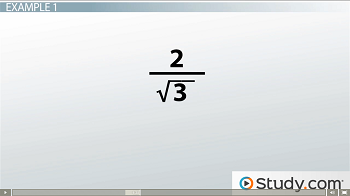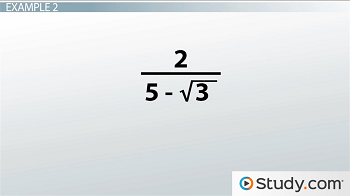# How to Rationalize the Denominator with a Radical Expression

An error occurred trying to load this video.

Try refreshing the page, or contact customer support.

Coming up next: Algebraic Numbers and Transcendental Numbers

### You're on a roll. Keep up the good work!

Replay
Your next lesson will play in 10 seconds
• 0:02 An Irrational Denominator
• 0:57 How to Rationalize the…
• 1:28 Example 1
• 2:16 Example 2
• 3:05 Lesson Summary

Want to watch this again later?

Timeline
Autoplay
Autoplay
Speed

#### Recommended Lessons and Courses for You

Lesson Transcript
Instructor: Yuanxin (Amy) Yang Alcocer

Amy has a master's degree in secondary education and has taught math at a public charter high school.

Watch this video lesson to learn what you can do to simplify your expression when you have a radical in the denominator. Learn what the single step solution to your problem is.

## An Irrational Denominator

What do you think of when you hear the word irrational? I think of things that don't make sense. It's like a friend of mine telling me this story where he saw a giant blue cartoon character walk down the aisle of the grocery store. I know that doesn't make sense. Well, in math, an irrational denominator is similar because it has a radical in the denominator, which doesn't make sense in a fraction. In math, fractions should always have a number in the denominator. It shouldn't have radicals in them. Recall that radicals are those numbers inside the symbol that is also used by the square root. The square root is a radical with an index of 2. Because fractions shouldn't have radicals in the denominator, whenever we see a radical in the denominator we rationalize the denominator, or move the radical to the numerator. It is perfectly okay for a fraction to have a radical in the numerator.

## How to Rationalize the Denominator

How do we rationalize the denominator? If our denominator only has a radical, to move this radical to the numerator, we multiply the numerator and denominator by the same radical.

However, if our denominator has another number plus or minus a radical, then we need to multiply the numerator and denominator by the same denominator but with the opposite sign in between the two terms. We call this multiplying by the conjugate.

Let's see an example of each now.

## Example 1In this first example, we have a denominator with a single radical.

Having this radical in the denominator makes our fraction not make sense, so we need to move it to the numerator. Because this is a single radical in the denominator, we can move it to the numerator by just multiplying both the numerator and denominator by the same radical. So, we multiply by the square root of 3 in the numerator and the denominator. We get 2 times the square root of 3 in the numerator, and we get a nice simple 3 in the denominator. Ah, now our fraction makes sense. We have rationalized our denominator. Because we can't simplify the square root of 3 further, we leave the numerator as 2 times the square root of 3.

## Example 2To unlock this lesson you must be a Study.com Member.

### Register to view this lesson

Are you a student or a teacher?

### Unlock Your Education

#### See for yourself why 30 million people use Study.com

##### Become a Study.com member and start learning now.
Back
What teachers are saying about Study.com

### Earning College Credit

Did you know… We have over 160 college courses that prepare you to earn credit by exam that is accepted by over 1,500 colleges and universities. You can test out of the first two years of college and save thousands off your degree. Anyone can earn credit-by-exam regardless of age or education level.